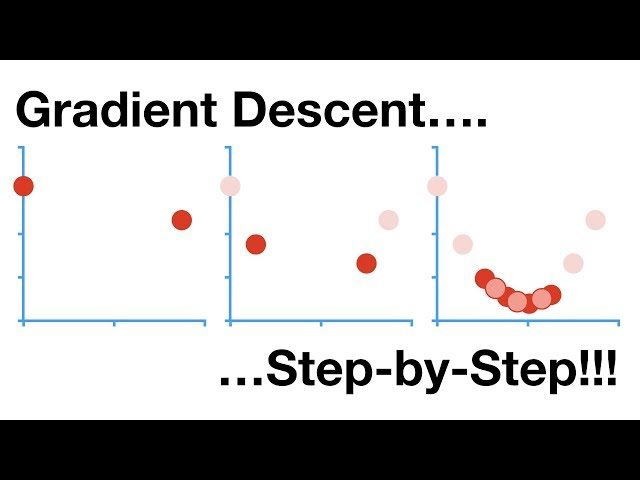# What is Numerical Optimization

#### The Importance of Numerical Optimization in AI

Numerical optimization is an essential component of artificial intelligence (AI), used to find the best solution to a given problem. Whether it is in machine learning, deep learning, robotics, or any other field of AI, numerical optimization plays a critical role in algorithm development, model training, and decision-making. In this article, we will explore the concepts of numerical optimization, the different techniques used, and their applications in AI.

#### What is Numerical Optimization?

Numerical optimization is a mathematical approach used to find the best value of a function (objective function) with respect to a set of parameters. The objective function may have multiple variables, and the goal of numerical optimization is to find the optimal set of values for these variables that will produce the best result (maximum or minimum) of the objective function. In other words, numerical optimization is about finding the optimal solution to a problem, given a set of constraints.

Numerical optimization is used in a variety of applications, ranging from finance, engineering, physics, and computer science. In AI, numerical optimization is used in various tasks such as:

• Regression and Classification: In supervised learning, numerical optimization is used to train machine learning models to minimize the loss function and predict the outcome of new data.
• Neural Network Training: In deep learning, numerical optimization is used to adjust the weights of a neural network to fit the input data and produce accurate output predictions.
• Reinforcement Learning: In unsupervised learning, numerical optimization is used to identify the optimal policy that maximizes the reward function in a dynamic environment.
• Robotics and Control Systems: Numerical optimization is used to optimize the control parameters of robots and other autonomous systems to achieve the desired behavior and performance.

#### Types of Numerical Optimization Techniques

There are many types of numerical optimization techniques available, each with its own strengths and weaknesses. Some of the most popular optimization techniques used in AI include:

• Gradient Descent: Gradient descent is a widely used optimization technique that iteratively adjusts the parameters of an objective function to minimize the cost function. It works by computing the gradient of the cost function with respect to the parameters and updating the parameters in the opposite direction of the gradient until the minimum is found.
• Stochastic Gradient Descent: Stochastic gradient descent is a variant of gradient descent that updates the parameters of the objective function using a randomly selected subset of the training data. It is faster and more efficient than gradient descent, especially for large datasets.
• Newton's Method: Newton's method is an optimization technique that uses the second derivative (Hessian) of the objective function to find the minimum. It is faster than gradient descent but requires more computational resources.
• Quasi-Newton Methods: Quasi-Newton methods are optimization techniques that approximate the Hessian matrix and use it to update the parameters of the objective function. They are faster than Newton's method and more stable than gradient descent.
• Conjugate Gradient Descent: Conjugate gradient descent is an optimization technique that updates the parameters of the objective function in a conjugate direction, which speeds up the convergence to the minimum.
• Genetic Algorithms: Genetic algorithms are optimization techniques inspired by natural selection. They use a population of candidate solutions that undergo mutation and crossover to produce better solutions over time.
• Particle Swarm Optimization: Particle swarm optimization is an optimization technique inspired by the swarming behavior of birds or fish. It uses a population of particles that interact with each other and move towards the optimal solution.
• Simulated Annealing: Simulated annealing is an optimization technique that mimics the annealing process of metals. It starts with a high temperature that gradually decreases over time, allowing the particles to escape local optima and converge to the global optimal solution.

#### Applications of Numerical Optimization in AI

Numerical optimization is applied in various areas of AI, including:

• Image and Speech Recognition: In image and speech recognition, numerical optimization is used to identify the features that are most useful for classification and to optimize the classification algorithm to produce accurate results.
• Natural Language Processing: In natural language processing, numerical optimization is used to optimize the weights of the neural network that maps the input text to the output sentiment or topic.
• Robotics: In robotics, numerical optimization is used to optimize the kinematics and dynamics of the robot to achieve the desired behavior and performance.
• Autonomous Driving: In autonomous driving, numerical optimization is used to optimize the path planning and control parameters of the vehicle to ensure safety and efficiency.
• Financial Analytics: In financial analytics, numerical optimization is used to optimize the portfolio of investments to maximize returns and minimize risks.

#### Conclusion

Numerical optimization is a critical component of AI, utilized to find the optimal solution to a given problem. There are many types of numerical optimization techniques available, each with its strengths and weaknesses. The application of numerical optimization in AI is widespread and ranges from machine learning and deep learning to robotics, autonomous systems, and financial analytics. Knowing the different numerical optimization techniques and their applications is essential for designing AI algorithms that are efficient, accurate, and robust.